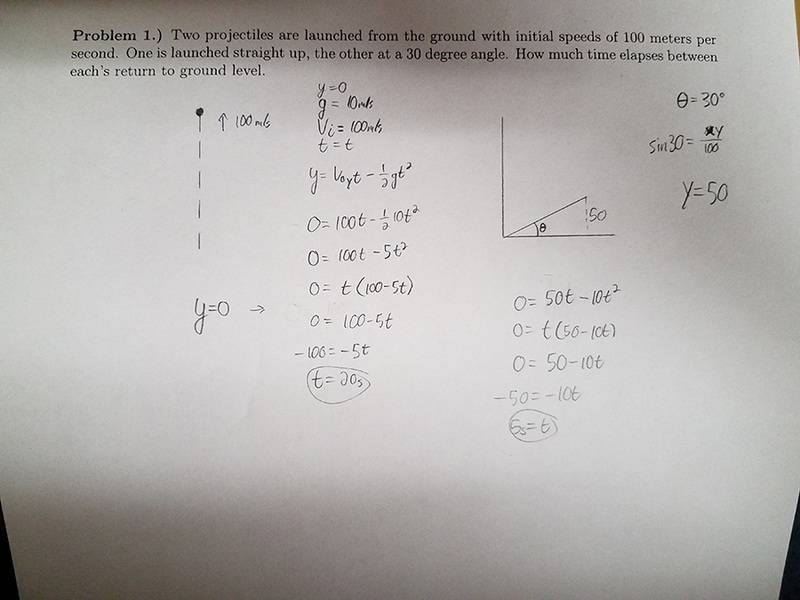# Finding Projectiles' Flight Times...

## Homework Statement

Two projectiles are launched from the ground with initial speeds of 100 meters per second. One is launched straight up, the other at a 30-degree angle. How much time elapses between each's return to ground level?

Theta = 30
g = 10 m/s
Vinitial = 100

y = vt - 1/2gt^2

## The Attempt at a Solution

As you can see in the image, I attempted to use the equations that I THINK were correct for this problem. My professor just threw a bunch of equations up on the board, some of which he said we don't need for now and they're just there to be there...so, hopefully I did these right.PeroK
Homework Helper
Gold Member
2020 Award
The attachment doesn't make your approach clear. How did you go about solving this?

And, what answer did you get?

The attachment doesn't make your approach clear. How did you go about solving this?

And, what answer did you get?
I wrote the formula that I used on the paper...it's right underneath the given variables. My answers were that the object thrown straight up in the air took 20 seconds to fall, and the object that was thrown at a 30 degree angle took 5 seconds to fall.

PeroK
Homework Helper
Gold Member
2020 Award
I wrote the formula that I used on the paper...it's right underneath the given variables. My answers were that the object thrown straight up in the air took 20 seconds to fall, and the object that was thrown at a 30 degree angle took 5 seconds to fall.
Why 5 seconds?

Why 5 seconds?
Because, after plugging in 0 for y (when the object hits the ground), I got the equation 0 = 50t-10t^2, and after solving for t, I got 5.

PeroK
Homework Helper
Gold Member
2020 Award
Because, after plugging in 0 for y (when the object hits the ground), I got the equation 0 = 50t-10t^2, and after solving for t, I got 5.

So, why 20 seconds in the first case?

PeroK
Homework Helper
Gold Member
2020 Award
So, why 20 seconds in the first case?

Don't you think the difference between 20 and 5 is too large?

You can view from vertical perspective.. from ground to max for each projectile.

First, vy=100.. using vt=v-gt
t=v/g=100/10=10s

Second, vy=100.sin30=50 m/s
t=5seconds

Total time for projectile 1 is 20s
Time for projectile 2 is 10s
So difference/time elapse is 20-10=10s